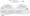# Data Structure Notes

Abstract Data Type

An abstract data type (ADT) is a set of data values and associated operations that are precisely specified independent of any particular implementation; such a data type is abstract in the sense that it is independent of various concrete implementations. Some of the examples of the ADT are String, List, Linked, Stack, Queue, Binary search tree, Priority queue and more.

Binary Tree

The simplest form of tree is a binary tree. A binary tree consists of  maximum two child nodes,
a. a node (called the root node) and
b. left and right sub-trees.
Both the sub-trees are themselves binary trees.Infix to Postfix Conversion

Converting Infix to Postfix

•    Read in infix string, one item at a time.

•    If the item is an operand add to the postfix string.

•    If the item is an operator push it on the stack if

•    The stack is empty

•    If the precedence of the item at the top of the stack is < the item being processed

•    If the precedence of the item at the top of the stack is > the item being processed pop it to the postfix string

•    When the infix string is empty pop the elements of the stack onto the postfix string to get the result

The Algorithm

1.    Initialize the stack by pushing a “(” — this serves to mark the beginning of the stack and give it an initial precedence. Append a “)” to the input to pop the initial “(” from the stack.

2.    If the input is empty, go to Finished.

3.    Read one token (operand, parenthesis, operator) from the input.

4.    If the token is an operand, output it and goto Step 1.

5.    Look up the precedence of the input token in the “Input” column of the precedence table.

6.    Look up the precedence of the token on top of the stack in the “Stack” column of the precedence table.

7.    If the precedence of the top of the stack is greater than or equal to the precedence of the input, first pop the token off the top of the stack. If it is not a “(”, output it. Either way, go to Step 5. Otherwise, if the precedence of the top of the stack is less than the precedence of the input, then if the input token is not a “)”, push it onto the stack

8.    Go to Step 1.

9.    Finished.